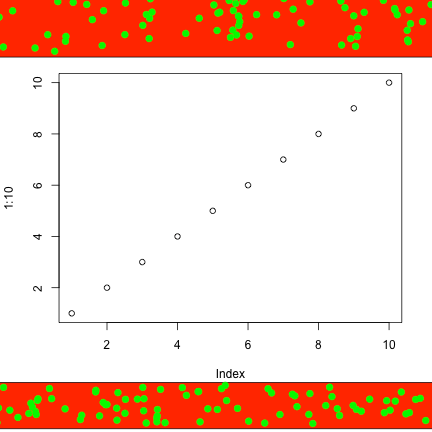Want to share your content on R-bloggers? click here if you have a blog, or here if you don't.

This is a guest post by Prasad Patil that answers the question: how to put a shape in the margin of an R plot?

The help page for R‘s `par()` function is a somewhat impenetrable list of abbreviations that allow you to manipulate anything and everything in the plotting device. You may have used this function in the past to create an array of plots (using `mfrow` or `mfcol`) or to set margins (`mar` or `mai`).

Way down toward the end of the list is the often-overlooked `xpd` parameter. This value specifies where in the plotting device an object can actually be plotted. The default is `xpd = FALSE`, which means that plotting is clipped, or restricted, to the plotting region. In other words, if your plot has `xlim = c(0, 10)` and `ylim = c(0, 10)` and you try to plot the point (-1, -1), it will not appear anywhere in the device.

`xpd` takes two other values, `TRUE` and `NA`, which limit plotting to the figure and device region, respectively. If you’re fuzzy on plotting terms, this tutorial presents those topics well.

## Plotting outside the plot

If you want to plot outside of the plotting region, I find that setting `xpd = NA` easiest since it opens up all external space. We also need to make sure that we keep space outside of the plot so that we have room to place our objects. Let’s say we want to put an ugly border above and below our plot:

```# Set xpd=NA and expand the top and bottom margins
par(xpd = NA, mar = par()\$mar + c(2.5, 0, 1, 0))
plot(1:10)
# Note that the rectangle we make here has corner coordinates outside of
# our plotting device
rect(-5, 11, 12, 14, col="red")
# Random dots in our rectangluar region
points(runif(100, -4.2, 12.8), runif(100, 11.2, 13.6), col = "green", pch = 19, cex = 1.2)
# And another rectangle for below
rect(-5, -1.7, 12, -3.5, col="red")
points(runif(100, -4.2, 12.8), runif(100, -3.3, -1.8), col = "green", pch = 19, cex = 1.2)```Here we mentally extend the axes of our plot to determine where to put our margin elements. One can imagine a diagonal for the top rectangle running from (-5,11) to (12,14). Neither of these points appear in the plot itself, but we used the established axes to estimate them and plot outside the plotting region.

## Images outside the plot

Now let’s say we want to add a logo or other external image in the margin of our plot. We will use R‘s `png` library to load a PNG image and `rasterImage()` to plot it:

```## If needed: install.packages("png")
library(png)
par(xpd = NA, mar=par()\$mar + c(3, 0, 0, 0))
plot(1:10)
rasterImage(img, 0.5, -2.5, 10.5, -1)```Here we used the `png` library and the `rasterImage()` command to read in and plot the "logo.png" file. Based on the previously-known dimensions of the logo, we can choose which points to use as endpoints for the image. Note that this image may appear stretched or contorted depending on the size of your R plot device, and it will not stay consistent if you resize.